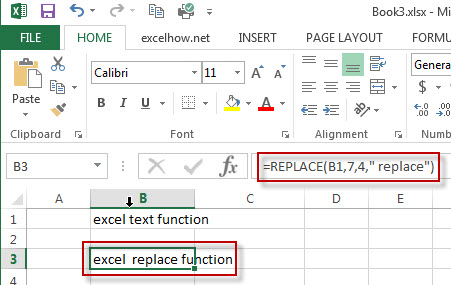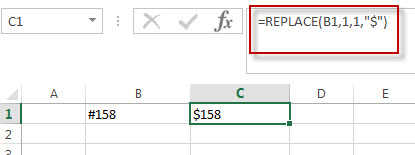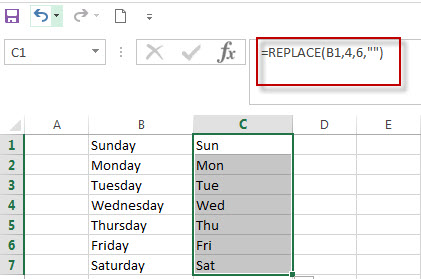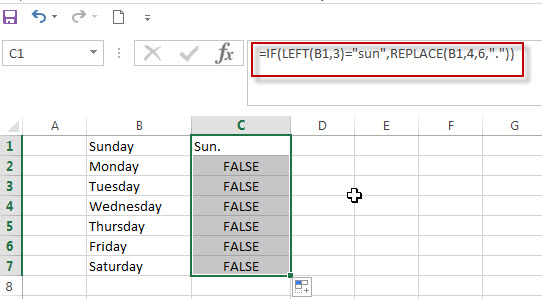# Excel Replace Function

This post will guide you how to use Excel REPLACE function with syntax and examples in Microsoft excel.

## Description

The Excel REPLACE function replaces all or part of a text string with another text string

The REPLACE function is a build-in function in Microsoft Excel and it is categorized as a Text Function.

The REPLACE function is available in Excel 2016, Excel 2013, Excel 2010, Excel 2007, Excel 2003, Excel XP, Excel 2000, Excel 2011 for Mac.

## Syntax

The syntax of the REPLACE function is as below:

`=REPLACE (old_text, start_num, num_chars, new_text)`

Where the REPLACE function arguments are:
Old_text -This is a required argument. The text string that you want to replace all or part of
Start_num – This is a required argument. The position of the first character that you want to replace within old_text.
Num_chars – This is a required argument. The number of characters that you want to replace within old_text
New_text – This is a required argument. The new text that will replace characters in old_text text string.

Note: The returned results of REPLACE function are treated as text string in EXCEL and if you are using the REPLACE function with numbers in calculations, the error message may be returned.

​There is another replace function called REPLACEB function. It is designed to work with double-type characters set. Such as: simplified Chinese.

## Excel REPLACE Function Examples

The below examples will show you how to use Excel REPLACE Text function to replace part of a text string with another new text string.

#1 To replace 4 characters in B1 cell with a new text string and starting with 7th character in old_text text, just using formula:

`=REPLACE(B1,7,4," replace")`#2 you can use the Excel’s REPLACE function to replace unwanted text string in a cell with another text string or empty string.

When you copied or imported data from the external application (such as: Microsoft word), it may include unwanted characters or text string along with the good data. At this time, you can use the REPLACE function to remove it.

For example, if you want to replace the first character of the text string “#158” with a dollar sign, you can use the below REPLACE formula:

`=REPLACE(B1,1,1,"\$")`You will get the result as: \$158 in the above formula.

## Related Functions

• Excel Replace function
The Excel DATE function returns the serial number for a date.The syntax of the DATE function is as below:= DATE (year, month, day) …
• Excel Substitute function
The Excel SUBSTITUTE function replaces a new text string for an old text string in a text string. ….
• Excel FIND function
The Excel FIND function returns the position of the first text string (substring) from the first character of the second text string.The FIND function is a build-in function in Microsoft Excel and it is categorized as a Text Function.The syntax of the FIND function is as below:= FIND  (find_text, within_text,[start_num])…

Question 1:

I have a number of cell that contains day name of the week, and I want to change the full day name of the week to the abbreviation of day name. How to achieve this requirement in excel.

Answer: you can use Text function or replace function to achieve it. I will show you how to use REPLACE function to change the full day name of the week, just entering the following formula to the cell B1,

`=REPLACE(B1,4,6,"")`Question2: I have a data in the excel worksheet, and I want to check if the first three characters are “sun” in a cell and then replace others characters with “.”, just keep “sun” characters in this cell. How to achieve this using excel formula.

Answer:  To achieve this, you need to use Replace function with IF function and LEFT function, using IF function to check the first characters if it is equal to “sun”, if TRUE, then call REPLACE function to remove the rest characters with “.”.  And Using LEFT function to get the first three characters of a cell.

So we can write down the following formula:

`=IF(LEFT(B1,3)="sun",REPLACE(B1,4,6,"."))`More reading: Using Find and Replace Tool to replace text in Excel